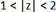# Let x[n]=(-1) n u[n-n 0 ] +an u[-n]. Determine the constraints on the complex number a and the…

Let x[n]=(−1)nu[n−n0]+αnu[−n]. Determine the constraints on the complex number α and the integer n0 so that the z trans form X(z) exists with region of convergence.